Next: Eigenstates and Eigenvalues Up: Fundamentals of Quantum Mechanics Previous: Momentum Representation

# Heisenberg's Uncertainty Principle

Consider a real-space Hermitian operator. A straightforward generalization of Eq. (193) yields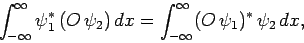(222)

where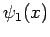andare general functions.

Let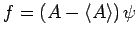, where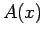is an Hermitian operator, and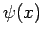a general wavefunction. We have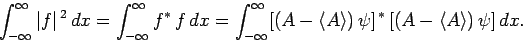(223)

Making use of Eq. (222), we obtain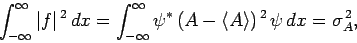(224)

whereis the variance of[see Eq. (160)]. Similarly, if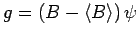, whereis a second Hermitian operator, then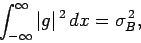(225)

Now, there is a standard result in mathematics, known as the Schwartz inequality, which states that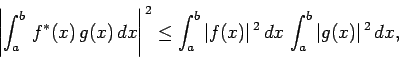(226)

where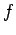and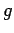are two general functions. Furthermore, ifis a complex number then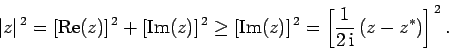(227)

Hence, if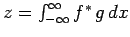then Eqs. (224)-(227) yield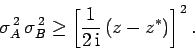(228)

However,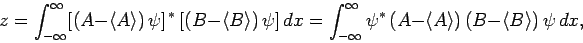(229)

where use has been made of Eq. (222). The above equation reduces to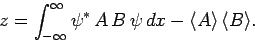(230)

Furthermore, it is easily demonstrated that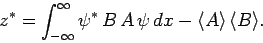(231)

Hence, Eq. (228) gives(232)

where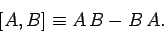(233)

Equation (232) is the general form of Heisenberg's uncertainty principle in quantum mechanics. It states that if two dynamical variables are represented by the two Hermitian operatorsand, and these operators do not commute (i.e.,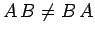), then it is impossible to simultaneously (exactly) measure the two variables. Instead, the product of the variances in the measurements is always greater than some critical value, which depends on the extent to which the two operators do not commute.

For instance, displacement and momentum are represented (in real-space) by the operatorsand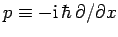, respectively. Now, it is easily demonstrated that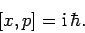(234)

Thus,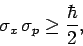(235)

which can be recognized as the standard displacement-momentum uncertainty principle (see Sect. 3.14). It turns out that the minimum uncertainty (i.e.,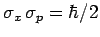) is only achieved by Gaussian wave packets (see Sect. 3.12): i.e.,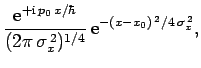(236)(237)

where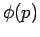is the momentum-space equivalent of.

Energy and time are represented by the operatorsand, respectively. These operators do not commute, indicating that energy and time cannot be measured simultaneously. In fact,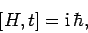(238)

so(239)

This can be written, somewhat less exactly, as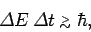(240)

whereand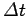are the uncertainties in energy and time, respectively. The above expression is generally known as the energy-time uncertainty principle.

For instance, suppose that a particle passes some fixed point on the-axis. Since the particle is, in reality, an extended wave packet, it takes a certain amount of timefor the particle to pass. Thus, there is an uncertainty,, in the arrival time of the particle. Moreover, since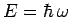, the only wavefunctions which have unique energies are those with unique frequencies: i.e., plane waves. Since a wave packet of finite extent is made up of a combination of plane waves of different wavenumbers, and, hence, different frequencies, there will be an uncertaintyin the particle's energy which is proportional to the range of frequencies of the plane waves making up the wave packet. The more compact the wave packet (and, hence, the smaller), the larger the range of frequencies of the constituent plane waves (and, hence, the large), and vice versa. To be more exact, if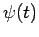is the wavefunction measured at the fixed point as a function of time, then we can write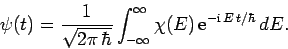(241)

In other words, we can expressas a linear combination of plane waves of definite energy. Here,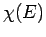is the complex amplitude of plane waves of energyin this combination. By Fourier's theorem, we also have(242)

For instance, ifis a Gaussian then it is easily shown thatis also a Gaussian: i.e.,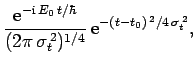(243)(244)

where. As before, Gaussian wave packets satisfy the minimum uncertainty principle. Conversely, non-Gaussian wave packets are characterized by.Next: Eigenstates and Eigenvalues Up: Fundamentals of Quantum Mechanics Previous: Momentum Representation
Richard Fitzpatrick 2010-07-20